# SSAT Middle Level Math : How to find range

## Example Questions

← Previous 1

### Example Question #3 : Find Measures Of Center, Variability, And Patterns In Data: Ccss.Math.Content.6.Sp.B.5c

The difference between 2996 and 4515 is closest to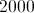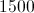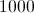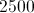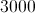Explanation:

Round 2996 up to 3000 and 4515 down to 4500.  The difference between 4500 and 3000 is 1500.

### Example Question #1 : Find Range

Find the range of this set of numbers:

89, 54, 76, 89, 94.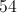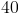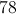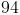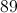Explanation:

First, order the numbers from least to greatest, then find the difference of the highest number and the lowest number.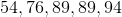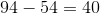Answer: The range of this set of numbers is 40.

### Example Question #2 : Find Range

Find the range of this set of numbers:

525, 436, 142, 213, 641.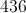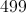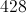Explanation:

First, order the numbers from least to greatest. Then, find the difference between the largest and the smallest number.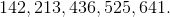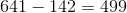Answer: The range is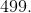### Example Question #3 : Find Range

What is the range of the set of numbers?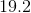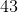Explanation:

The range of a set of numbers is the difference between the largest and smallest numbers. In this set,is the largest number andis the smallest number. The range, or difference, between these two numbers, is.

### Example Question #1 : How To Find Range

Find the range of this set of numbers: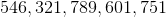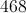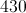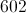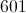Explanation:

First order the numbers in order from least to greatest: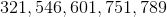Then, find the difference of the largest and smallest number in the set: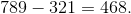Answer: The range is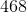### Example Question #5 : Find Range

What is the range of the set of numbers: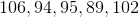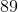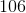Explanation:

The ragne of a set of numbers is the difference between the largest and smallest numbers. In this set,is the largest number andis the smallest number. The range, or difference, between these two numbers, is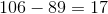.

### Example Question #6 : Find Range

What is the range of the following data set?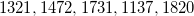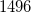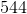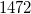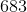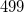Explanation:

The range of a data set is equal to the difference between the largest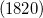and the smallest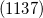numbers.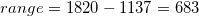### Example Question #7 : Find Range

Find the range of this set of numbers: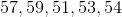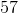Explanation:

First order the numbers from least to greatest: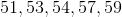Then subtract the smallest number from the largest number: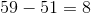Answer:### Example Question #1 : How To Find Range

Given the following set of numbers, find the range.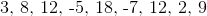Explanation:

In order to find the range for a set of data, take the largest number and subtract the smallest number.

In this case, the largest number isand the smallest number isRemember that when we subtract a negative, we are actually adding the absolute value of the numbers:

Therefore: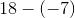is the same as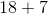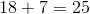### Example Question #1 : How To Find Range

Use the following data set to answer the question: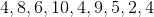Find the range.Explanation:

To find the range, we will find the smallest number and the largest number.  Then, we will find the difference of those two numbers.

So, given the setwe can see the smallest number is 2 and the largest number is 10.  Now, we will find the difference.  We get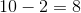Therefore, the range of the data set is 8.

← Previous 1